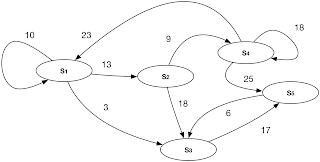## Thursday, 14 January 2016

### Using probability to predict state of a system

Although we can discuss this topic from mathematical point of view and specifically Markov process model but it is not that much difficult to talk about it in plain english too. What I want to talk about it is that we can use what we've talked about during last 2 or 3 posts to build a network of information to find out the most probable state of a system or process.

Suppose you have a system which at any give time could be in one the following elements of the state set:

S = { S1, S2, S3, ... , Sn }

We start monitoring the system and record its state-change in every monitoring interval, after a while we will have something like the following:Sample state transition network with transition score

Here it shows that we have seen 10 times transition from S1 to S1,  or 13 times transition from S1 to S2, ... or 25 times transition from S4 to S5 and ... We can easily convert these transition counts or scores to probability values. For example when your current state is S1 then you only have 3 options to go to next state, staying in S1 or going to S2 or going to S3. Each of these transitions has a probability which can be calculated as bellow:

P(S1 to S1) = 10 / (10+13+3) = 10 / 26
P(S1 to S2) = 13 / (10+13+3) = 13 / 26
P(S1 to S3) = 3 / (10+13+3) =  3 / 26

So it simply shows if you are in state S1 the most next probable state will be S2 then S1 and finally S3. Our monitoring or learning shows while you are in S1 there is no chance to be in any other states than these three. As you see just after a while of monitoring the system and transforming its state to a Bayesian Network like graph, we can have a graph which clearly shows the probability of system's next state based on the current state.

The only thing we need to keep in mind is an implicit assumption we took, which is the next state of the system is only depends on the current state. This is not in fact something odd, because whatever else the next state depends on, you can always consider it as some part of the current state. So you always should consider the state of the system as a vector consist of any variables which completely defines the current state and you require to define the next state based on them. If the next state somehow has some relation to a parameter from previous states you can have that parameter in current state too. This assumption is also has been taken in a Markov Process Model so we can say:

St+1 = F(St

Which means state of the system at time t+1 is just a function of its state at time t. Just note that F  is not an ordinary function it works on vectors, you can somehow consider it as some high dimension matrix or tensor of course, we will talk about it pretty soon and better than that, we will bring some easy to digest examples on how we can predict the future state of a system in practice.